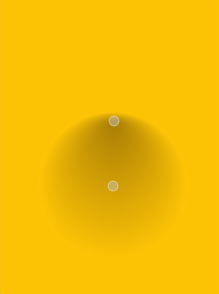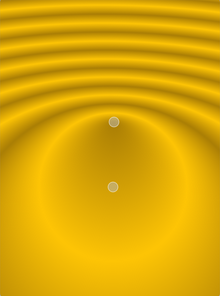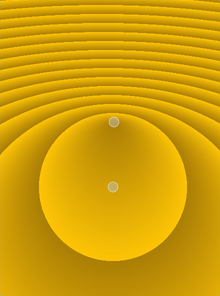## Detailed Description

Simple radial gradients interpolate colors between a focal point and end points on a circle surrounding it. Extended radial gradients interpolate colors between a focal circle and a center circle. Points outside the cone defined by the two circles will be transparent. For simple radial gradients the focal point is adjusted to lie inside the center circle, whereas the focal point can have any position in an extended radial gradient.

Outside the end points the gradient is either padded, reflected or repeated depending on the currently set spread method:PadSpread (default) ReflectSpread RepeatSpread

The colors in a gradient is defined using stop points of the QGradientStop type, i.e. a position and a color. Use the QGradient::setColorAt() or the QGradient::setStops() function to define the stop points. It is the gradient's complete set of stop points that describes how the gradient area should be filled. If no stop points have been specified, a gradient of black at 0 to white at 1 is used.

## Member Function Documentation

Constructs a simple radial gradient with the center at (cx, cy) and the specified radius. The focal point lies at the center of the circle.

Constructs a simple radial gradient with the given center, radius and the focal point in the circle center.

Constructs a simple radial gradient with the given center (cx, cy), radius and focal point (fx, fy).

Note: If the given focal point is outside the circle defined by the center (cx, cy) and the radius it will be re-adjusted to the intersection between the line from the center to the focal point and the circle.

Note: If the given focal point is outside the circle defined by the center point and radius, it will be re-adjusted to lie at a point on the circle where it intersects with the line from center to focalPoint.

Constructs a simple radial gradient with the center and focal point at (0, 0) with a radius of 1.

Sets the center of this radial gradient in logical coordinates to center.

Sets the center of this radial gradient in logical coordinates to (x, y).

Sets the focal point of this radial gradient in logical coordinates to focalPoint.

Sets the focal point of this radial gradient in logical coordinates to (x, y).